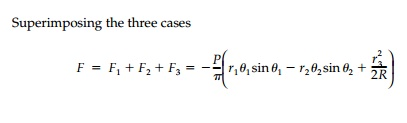Home | | Mechanics of Solids | Line Loads within the Half Space and Diametric Loading of a Circular Disk

## Chapter: Civil : Principles of Solid Mechanics : Wedges and the Half-Space

To complete our repertoire of 2D solutions for the half-space, we need a solution for a vertical and horizontal line load acting at some depth, d, below the surface of a semi-infinite mass.

Line Loads within the Half Space

To complete our repertoire of 2D solutions for the half-space, we need a solution for a vertical and horizontal line load acting at some depth, d, below the surface of a semi-infinite mass. Referring to Figure 8.8, the solution for plane strain*This solution is again very useful for numerical analysis. It is important to recognize that Poisson’s ratio now appears in what would seem to be a two-dimensional problem. This is due to the displacement conditions in the far field which we hypothesize is probably the cause of difficulties with some of the previous wedge solutions. We will return to this question of existence and uniqueness in the concluding section of this chapter after the solution for constant body force loading of wedges is presented.

It is somewhat surprising that wedge solutions can be applied to determine stresses in disks under self-equilibrating loads. The simplest example is a disk with diametric, concentrated loads as shown in Figure 8.9.*We can then remove this pressure to obtain the final result. More specifically, if we associate the stress function F1 with the top load and F2 with the bottom load then:Finally, applying a uniform boundary tension from the Lamé solution with the origin at the center of the diskgiving the stresses at any point as the sum of three states, which must be transformed to a common orientation. For example, at the center of the disk whereThe distribution of vertical stress along the horizontal diameter given by the expressionStudy Material, Lecturing Notes, Assignment, Reference, Wiki description explanation, brief detail
Civil : Principles of Solid Mechanics : Wedges and the Half-Space : Line Loads within the Half Space and Diametric Loading of a Circular Disk |

Related Topics